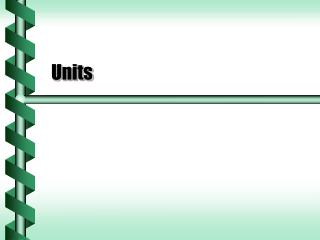DownloadDownload PresentationUnits

Units

Download PresentationUnits

- - - - - - - - - - - - - - - - - - - - - - - - - - - E N D - - - - - - - - - - - - - - - - - - - - - - - - - - -
Presentation Transcript

1. Units

2. Unit Systems • Systems set up fundamental units. • British system - foot, pound, second • Metric system - meter, kilogram, second

3. Time • The unit of time originally was based on the day and the year. • The second was 1/60 * 1/60 *1/24 of a day. • In the 20th century the second was measured based on the timing of atoms. • We now know that the day is getting longer and “leap seconds” are added every few years. • The SI unit of time is the second (s)

4. Length • The oldest standards of length were based on the human body. • The metric system defined the meter in terms of the Earth: 1/10,000,000 from the pole to the equator. • The meter is now defined in terms of the second and speed of light. • The SI unit of length is the meter (m)

5. Mass • Standard weights have been maintained for centuries. • Weight and mass were thought to be the same. • Now a standard 1 kilogram mass is kept in Paris. • The SI unit of mass is the kilogram (kg)

6. Prefixes on units are used to represent powers of ten. Prefixes denote powers of ten from -18 to +18 in steps of three. Example: a kilometer is 103 meters or 1000 meters. Most Common micro () 10-6 milli (m) 10-3 kilo (k) 103 mega (M) 106 Common, but the power is not a factor of three. centi (c ) 10-2 deci (d ) 10-1 SI Prefixes

7. There are other fundamental units in SI. See NIST. ampere (A) kelvin (K) mole (mol) candela (cd) Derived units are built from the fundamental units. area (m2) volume (m3) velocity (m/s) acceleration (m/s2) force (kg m/s2) or (N) energy (kg m2/s2) or (J) Other Units

8. Density • Matter has mass and takes up volume. • The ratio of the mass to the volume is the density. Salt (solid): 2.165 x 103 kg/m3 Water (liquid): 1.000 x 103 kg/m3 Nitrogen (gas): 1.251 kg/m3

9. Matching Units • Conversion between units must be of the same type. • Length conversion: • 1 in = 2.54 cm • Time conversion: • 1 hr = 3.6 x 103 s • No conversion between different types of units. • 1 in is not equivalent to some seconds

10. Conversion Factors • A value is converted by applying the ratio of the conversion factors. • How many inches in 50. cm? • 50. cm (1 in / 2.54 cm) = (50. / 2.54) in = 20. in • Many conversion factors use scientific notation. • How many seconds in a year? • 1 yr (365 d/yr) (24 hr/d) (3.6 x 103 s/hr) = 31500 x 103 s = 3.15 x 107 s next